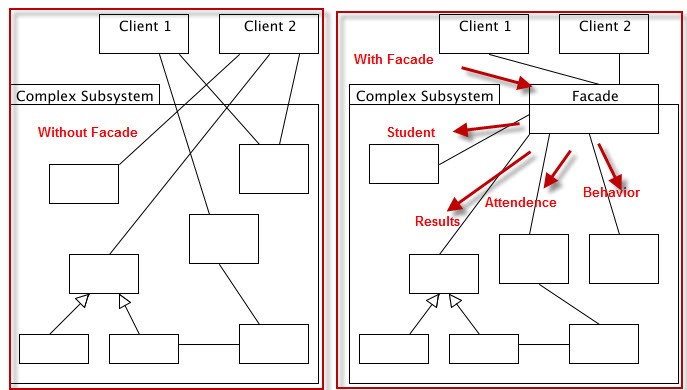• What is Facade design pattern?
• What are the basic principles?
• How to implement
• where we can use this.

I have used Java with Eclipse and C# with VS2010.

# What is Facade design patter?

Facade provides a simplified interface that performs many actions.

It is very easy and simple design that we may use every day and might not know.

Think of doing many task from different object, and if you keep all the working object in a class and call all the function using that class. This is the main them of facade design pattern.

Suppose, in a baking system, we have to withdraw 50 taka from our account. So, we have to perform

1. Account Information Validation Check( account number, pin number)
2. Withdrawing amount validation( whether I have money in the bank or not)
3. Withdraw the amount. So, in here if we use a separate class do all the task and give you result , then it is act as facade.

Facade pattern is a simplified version of moderator design pattern where only one active moderator class and the colleagues are passive classes.

# Principle :

• A class is responsible for certain duties involving with many other classes and functions.

# Concept Image# How to implement?

As we know, it is actually do the task with other objects and provide client a easy way to access those classes, so a class will be acting as facade.

And there will be task involving classes

And, there will be a client who actually call the facade to perform the task.

# Example :

Lets think about a school result publish system. As if we need to publish a student’s results to his parents as result card.

And, usually in the result card there are attendance percentage, behavior marking, subject marking etc are there.

So, let assume this three should be added in the result card along with date of birth and name.

So, here is the Student class contains student name and date of birth.

``````public class Student {
private String name = "Shantonu";
private String dateOfBirth = "5 jan 1986";
public String getName() {
return name;
}
public void setName(String name) {
this.name = name;
}
public String getDateOfBirth() {
return dateOfBirth;
}
public void setDateOfBirth(String dateOfBirth) {
this.dateOfBirth = dateOfBirth;
}
}
``````

And result , behavior and attendance class.

``````public class Results {
private Student student;
private double totalMarks = 1000.00;
public Results(Student aStudent){
student=aStudent;
}
public void getStudentResult(){
System.out.println(student.getName()+"'s Result = "+totalMarks+" Marks");
}
}
public class Behavior {
private Student student;
private String behavior = "Very Polite";
public Behavior(Student aStudent){
student=aStudent;
}
public void getStudentBehavior(){
System.out.println(student.getName()+"'s behavior is "+behavior);
}
}
public class Attendance {
private Student student;
private double attendance = 85.6;
public Attendance(Student aStudent){
student=aStudent;
}
public void getStudentAttendance(){
System.out.println(student.getName()+"'s attendance = "+attendance+" percentage");
}
}
``````

Now, we will implement all function together in a facade class. Let’s call it ReportCardFacade

``````public class ReportCardFacade {
private Student student;
private Results studentResult;
private Behavior studentBehavior;
private Attendance studentAttendance;
student=aStudent;
studentResult = new Results(aStudent);
studentBehavior=new Behavior(aStudent);
studentAttendance=new Attendance(aStudent);
}
public void getResultCard() {
System.out.println("This is result of "+student.getName()+" Who's date of birth is "+student.getDateOfBirth());
studentResult.getStudentResult();
studentAttendance.getStudentAttendance();
studentBehavior.getStudentBehavior();
}
}
``````

And finally, facade calling client who contain the main program.

``````public class Program {
public static void main(String[] args) {
Student aSudent = new Student();